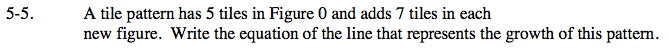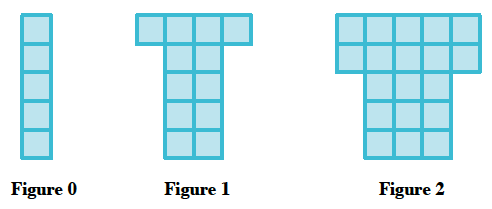Home > CAAC > Chapter 5 > Lesson 5.1.1 > Problem5-5

5-5.

A tile pattern has 5 tiles in Figure 0 and adds 7 tiles in each new figure. Write the equation of the line that represents the growth of this pattern. 5-5 HW eTool (Desmos). Homework Help ✎Illustrate the problem.Use the equation of a line, y = mx + b, to substitute in known information.

y = 7x + 5

Use the eTool below to help you with this problem.
Click on the link at right for the full eTool version: AC 5-5 HW eTool